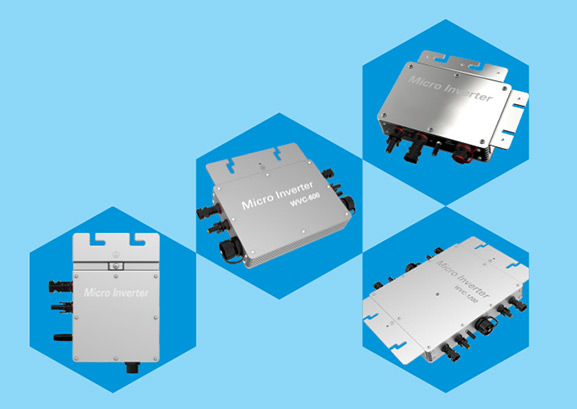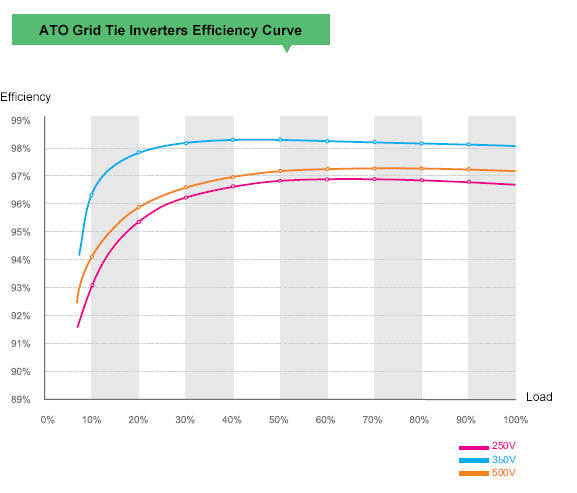# How Does Input Voltage Affect a Grid-Tie Inverter?

In the photovoltaic grid-tie inverter, there are many input voltage technical parameters: Maximum DC input voltage, MPPT operating voltage range, full-load voltage range, start-up voltage, rated input voltage and so on. These parameters have their own focus and all of them are useful.

Maximum DC input voltage

The maximum DC input voltage is to restrict the maximum open-circuit voltage of the string. It is required that the maximum open circuit voltage of the string cannot exceed the maximum DC input voltage at the lowest limit temperature. For example, the open-circuit voltage of the component is 38V and the temperature coefficient is -0.3%/°C, at minus 25 °C, the open-circuit voltage is 43.7V, then the maximum number of strings is 25. 25*43.7=1092.5V.

The maximum DC input voltage is a little higher than the MPPT operating maximum voltage. The start-up voltage is higher than the MPPT operating minimum voltage. This is because the maximum DC input voltage and the start-up voltage are two parameters corresponding to the open-circuit state of the component. Moreover, the open-circuit voltage of the component is generally about 20% higher than the working voltage.

MPPT operating voltage range

MPPT operating voltage range is designed for the grid tie inverter to adapt to the changing voltages of the component. The voltage of the component changes according to the changes of illumination and temperature. The number of components connected in series is also designed according to the specific conditions of the project, so the inverter is set with a working range. As long as it is within this range, the on grid inverter can always work normally. If the voltage scope is wider, it means that the applicability of the inverter is wider.The full-load voltage range is that the inverter can output the rated power within this voltage range. It means that, in addition to the PV module, there are some other applications of the inverter. The inverter has a maximum input current, such as 40A for 40kW. Only when the input voltage exceeds 550V, the output is likely to reach 40kW. When the input voltage exceeds 800V, the heat generated by the loss increases sharply, causing the inverter to derate the output. Therefore, the string voltage should be designed in the middle of the full load voltage range as much as possible .

Start-up voltage

Before the power inverter starts, the component does not work and it is in the open state, the voltage will be relatively high. When the inverter starts, the component is in working state and the voltage will decrease. In order to prevent the inverter from being started repeatedly, the start-up voltage of the inverter is higher than the minimum operating voltage. After the grid tie inverter is started, it does not mean that the inverter will have power output immediately. The control part of the on grid inverter, the CPU and the screen and other devices work first. Firstly, the inverter inspects itself, and then the component and the power grid are detected. When there is completely no problem, the inverter will have an output if the photovoltaic power exceeds the standby power of the inverter.

Rated input voltage

Please remember that it is rather difficult to have several parameters for the power inverter. In the design of photovoltaic system, as a matter of fact, remembering the "rated input voltage" parameter is once for all. It can automatically meet other technical parameters. The secret is in the following diagram:This figure has 3 lines, representing the efficiency of the grid tie inverter when there are three kinds of input voltages. It is visible that that different voltages have different efficiency. Among them, the blue line 360V has the highest efficiency, followed by red line 500V, and the purple line 250V has the lowest efficiency. This conveys the message that if the voltage of the string is designed around the rated voltage, the efficiency of the inverter will be very high and the power generation capacity will be high.

The reason for this starts from the principle of the power inverter. For the DC-DC-BOOST circuit of the string inverter, the DC voltage needs to be boosted and stabilized to a certain value (this is called the DC bus voltage) before it can be converted to AC power. As to the 230V output, its DC bus voltage should be about 360V. As to the 400V output, its DC bus voltage should be about 600V. As to the 500V output, its DC bus voltage should be about 750V. As to the 540V output, its DC bus voltage should be about 800V. However, the component series connected voltage is generally not so high, and the circuit needs to be adjusted. The grid tie inverter is generally adjusted by PWM. There is a term called duty ratio, which is equal to the component series voltage/ DC bus voltage. The duty ratio has a close relationship with the efficiency. A higher duty ratio tends to generate smaller voltage difference and higher efficiency.

If you master this secret, the component with the power inverter does not need to calculate complex formulas. Just try to match the string voltage to the rated operating voltage of the inverter, you will have the highest efficiency, and the maximum voltage will not be exceeded at the extreme low temperature. It will also be within the full load MPPT voltage range during operation, which is absolutely simple and practical. Take 60 pieces of 280W polycrystalline components as an example, single-phase 220V inverter with the input rated voltage of 360V, equipping with 11 and 12 components will have the best effect. Three-phase 400V inverter with the input rated voltage of 600V, equipping with 20 and 21 components will have the best effect. Three-phase 480V power inverter with the input rated voltage of 700V, equipping with 23 and 24 components will have the best effect. If this condition is not met, you can try to get close to this voltage as much as possible.